How to perform data validation using python by processing only matched columns?
BIG DATA RECIPES DATA CLEANING PYTHON DATA MUNGING MACHINE LEARNING RECIPES PANDAS CHEATSHEET     ALL TAGS

# How to perform data validation using python by processing only matched columns?

This recipe helps you perform data validation using python by processing only matched columns

0

## Recipe Objective

In most of the big data scenarios , Data validation is checking the accuracy and quality of source data before using, importing or otherwise processing data. Different types of validation can be performed depending on destination constraints or objectives. Data validation is a form of data cleansing. In most of the production environments , data validation is a key step in data pipelines.

## System requirements :

• Install the python module as follows if the below modules are not found:
• `pip install pandas`
• The below codes can be run in Jupyter notebook, or any python console

## Step 1: Import the module

In this scenario we are going to use pandas numpy and random libraries import the libraries as below :

``` import pandas as pd ```

## Step 2 :Prepare the dataset

Data of Output looks as follows: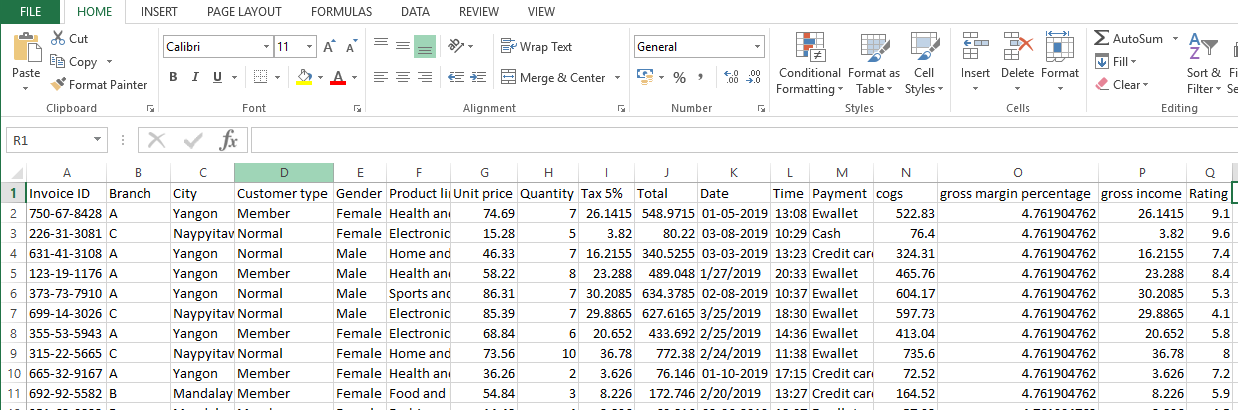## Step 3: Validate the data frame

To validate the data frame is empty or not using below code as follows :

``` def read_file(): df = pd.read_csv(filename) if(df.empty): print ('CSV file is empty') else: print ('CSV file is not empty') return df ```

Pass the file name as the argument as below :

``` filename ='C:\\Users\\nfinity\\Downloads\\Data sets\\supermarket_sales.csv' ```

Call the function as belows is

``` df = read_file() ```

Output of the above code :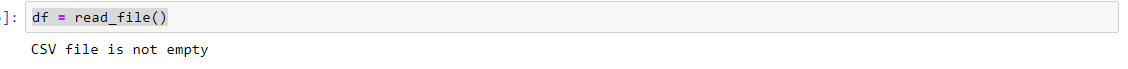Using pandas library to determine the csv data datatype by iterating the rows :

``` import pandas as pd df = pd.read_csv(supermarket_sales.csv', nrows=2) for dtype in df.dtypes.iteritems(): print(dtype) ```

Or also we can easily know the data types by using below code :

``` df.types ```

Output of the above lines :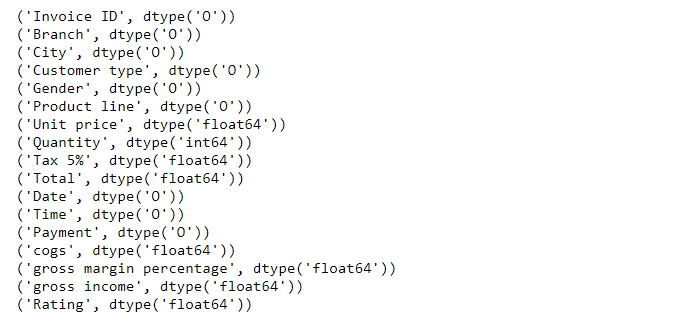## Step 4: Processing the matched columns

Here in this scenario we are going to processing only matched columns between validation and input data arrange the columns based on the column name as below.

``` import pandas as pd data = pd.read_csv('C:\\Users\\nfinity\\Downloads\\Data sets\\supermarket_sales.csv') df = df[sorted(data)] validation = df validation['chk'] = validation['Invoice ID'].apply(lambda x: True if x in df else False) validation = validation[validation['chk'] == True].reset_index() df ```

Output of the above code :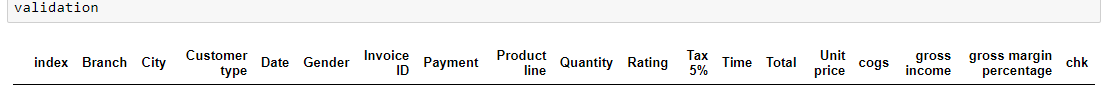## Step 5: Check Data Type convert as Date column

Here in this scenario we are going to check the columns data types and and convert the date column as below code:

``` for col in df.columns: if df[col].dtype == 'object': try: df[col] = pd.to_datetime(df[col]) except ValueError: pass print(df.dtypes) ```

Output of the above code:

``` renamed_data['buy_date'] = pd.to_datetime(renamed_data['buy_date']) renamed_data['buy_date'].head() ```

Output of the above code :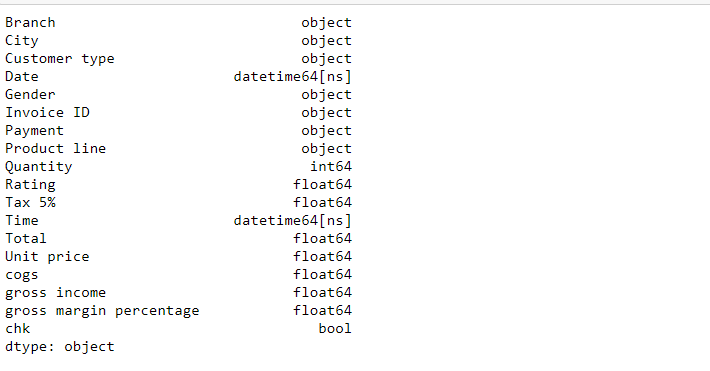## Step 6: validate data to check missing values

Here we are going to validating the data to checking the missing values, below code will loop the data column values and check if the columns has any missing value is as follow below

``` for col in df.columns: miss = df[col].isnull().sum() if miss>0: print("{} has {} missing value(s)".format(col,miss)) else: print("{} has NO missing value!".format(col)) ```

Output of the above code :#### Relevant Projects

##### Yelp Data Processing Using Spark And Hive Part 1
In this big data project, we will continue from a previous hive project "Data engineering on Yelp Datasets using Hadoop tools" and do the entire data processing using spark.

##### Build a big data pipeline with AWS Quicksight, Druid, and Hive
Use the dataset on aviation for analytics to simulate a complex real-world big data pipeline based on messaging with AWS Quicksight, Druid, NiFi, Kafka, and Hive.

##### Real-Time Log Processing in Kafka for Streaming Architecture
The goal of this apache kafka project is to process log entries from applications in real-time using Kafka for the streaming architecture in a microservice sense.

##### Event Data Analysis using AWS ELK Stack
This Elasticsearch example deploys the AWS ELK stack to analyse streaming event data. Tools used include Nifi, PySpark, Elasticsearch, Logstash and Kibana for visualisation.

##### Hadoop Project-Analysis of Yelp Dataset using Hadoop Hive
The goal of this hadoop project is to apply some data engineering principles to Yelp Dataset in the areas of processing, storage, and retrieval.

##### Online Hadoop Projects -Solving small file problem in Hadoop
In this hadoop project, we are going to be continuing the series on data engineering by discussing and implementing various ways to solve the hadoop small file problem.

##### Create A Data Pipeline Based On Messaging Using PySpark And Hive - Covid-19 Analysis
In this PySpark project, you will simulate a complex real-world data pipeline based on messaging. This project is deployed using the following tech stack - NiFi, PySpark, Hive, HDFS, Kafka, Airflow, Tableau and AWS QuickSight.

##### Tough engineering choices with large datasets in Hive Part - 1
Explore hive usage efficiently in this hadoop hive project using various file formats such as JSON, CSV, ORC, AVRO and compare their relative performances

##### Tough engineering choices with large datasets in Hive Part - 2
This is in continuation of the previous Hive project "Tough engineering choices with large datasets in Hive Part - 1", where we will work on processing big data sets using Hive.

##### Movielens dataset analysis for movie recommendations using Spark in Azure
In this Databricks Azure tutorial project, you will use Spark Sql to analyse the movielens dataset to provide movie recommendations. As part of this you will deploy Azure data factory, data pipelines and visualise the analysis.